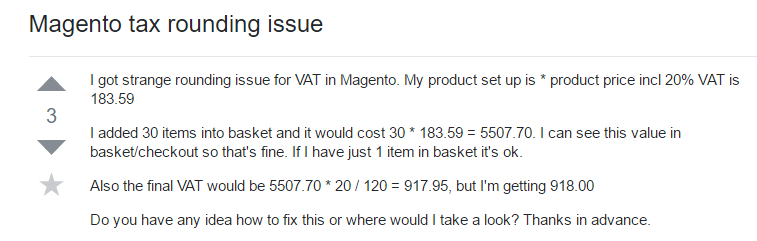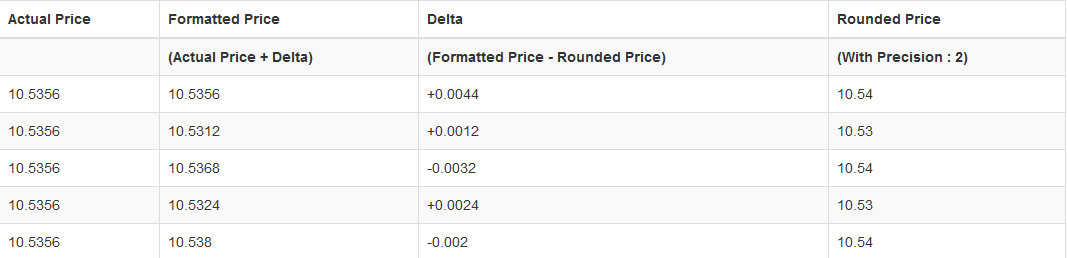# Magento Price Rounding

In e-commerce applications it is important to know how the prices are computed and what rules you need to apply in order to display the correct price. This article will present the Magento way of solving the round operations on prices and offer a view on the importance of price computations.

The reason why we need to be careful when computing prices or calculating totals is the type of data used to store prices, which in this case is float type. The float type in PHP uses the IEEE 754 standard, which depends on the platform. This dependency on the platform can result in a small possibility of getting different results for the same operations. More about the floating point numbers and arithmetic can be found in this article.

In order to understand the situation, we’ve started looking at the PHP documentation for float type and we’ve discovered a warning regarding this type:

"Floating point numbers have limited precision. Although it depends on the system, PHP typically uses the IEEE 754 double precision format, which will give a maximum relative error due to rounding in the order of 1.11e-16. Non elementary arithmetic operations may give larger errors, and, of course, error propagation must be considered when several operations are compounded."

And they’ve concluded with this:

"So never trust floating number results to the last digit, and do not compare floating point numbers directly for equality. If higher precision is necessary, the arbitrary precision math functions and gmp functions are available."

This type of warning makes us look twice at the methods used to compute prices. We would like to avoid situations like the ones described in this article.

The good part for the Magento community is that there was no incident reported about this kind of problem, or at least not one that I am aware of. But this doesn’t mean that we should not be careful with this.

## The Challenge

So what is the ugly part of the float type? Rounding operations made with the floating point type. Floating types are used to store numbers with high precision, but prices do not need a higher precision like number PI. The precision generally used for prices is of 2 digits, maximum 4 digits.

An exact situation that creates problems with rounding operations is this one:

• we have one product price - 98.10;

• the discount percentage is 55% and the discount price value is 53.96;

• the total value is 44.14 but we receive 44.15.

The PHP code that has been used to calculate the wrong total price is this:

``` \$discountPrice = (\$productPrice * \$discount) /100;
\$totalPrice = \$productPrice - \$discountPrice ;
return round(\$totalPrice, 2);
```

If we run that code, the result will be 44.15, not 44.14. If we investigate the total price before rounding, we’ll see the value 14.145.

What went wrong with that code? The discount price was not rounded to the same precision as the total price when it was used in the total price operation. This is the correct code:

``` \$discountPrice = round((\$productPrice * \$discount) /100, 2);
\$totalPrice = \$productPrice - \$discountPrice ;
return round(\$totalPrice, 2);
```

The takeaway from this situation is that we need to be careful when to put round operations in computing prices. We need to avoid situations like the one mentioned above.

## How does Magento 1 solve the rounding problems?

Before mentioning the classes and methods used by Magento for rounding, we need to understand how the price data is stored and displayed. By default, Magento sets prices in MySQL DB as decimal type with a precision of 4 digits. This means that the price stored looks like this - 98.1000. When the price is displayed, this precision of 4 digits is rounded to 2 digits. The above price will be displayed like this - 98.10.

From the above PHP code example, we have learned that operations with different precisions can cause problems and, of course, this also happens in Magento. Please check this post on stackoverflow.com:One solution recommended for that issue is a rewrite onthe Mage_Core_Model_Store::roundPrice method, where the precision was increased from 2 to 4, making the rounding operations be of the same precision. But that solution led to other problems, like processing payments with PayPal.

Magento uses the PHP round function to a precision of 2 when computing prices in totals, tax and discount operations. The method used for rounding is located in class Mage_Core_Model_Store and has the following signature:

```public function roundPrice(\$price);
```

We found another type of operation used by Magento to round and it’s called delta round. The deltaRound method can be found in the Mage_Core_Model_Calculator class and has the following implementation:

```public function deltaRound(\$price, \$negative = false)
{
\$roundedPrice = \$price;
if (\$roundedPrice) {
if (\$negative) {
\$this->_delta = -\$this->_delta;
}
\$price  += \$this->_delta;
\$roundedPrice = \$this->_store->roundPrice(\$price);
\$this->_delta = \$price - \$roundedPrice;
if (\$negative) {
\$this->_delta = -\$this->_delta;
}
}
return \$roundedPrice;
}
```

Based on that code, the steps that Magento takes for the rounding delta algorithm are:

• set a variable called delta where you keep a small float number like 0.000001 or 0.0;

• add the delta variable to the input price;

• round up the computed price;

• recalculate and store delta, whose value is the difference between the computed and the initial price.

The \$negative parameter will compute the delta for negative numbers but the prices are generally positive numbers. Negative prices are usually used for refunds or discounts. The delta round method is also declared in the next classes:

• Mage_Tax_Model_Sales_Total_Quote_Subtotal

• Mage_Tax_Model_Sales_Total_Quote_Tax

Because the rounding algorithm has impact on tax prices, we need to see how it’s used. First, we need to understand the main operation of the tax quote class. In that class there are 3 methods to calculate tax on the shopping cart:

• on total - it applies tax only on calculated totals;

• on row - it’s applied to individual product item price that is obtained from base product price multiplied with quantity;

• on unit - it’s applied to individual product item and adds everything together to get the tax rate.

The difference between unit base tax calculation and base row tax calculation is that the cart item price is not multiplied by quantity. The item price is calculated through many add operations.

The method used to calculate the tax can be set from admin:

• System -> Configuration -> Tax -> Calculation Settings;

• field name "Tax Calculation Method Based On".

The calculated tax based on item unit is the only method that uses the delta round algorithm. The following image shows how delta is computed for a product price of 10.5356 with 5 quantities in the cart (thanks to Rajeev K Tomy):The total price for that item will be:

10.54+10.53+10.54+10.53+10.54 = 52.68

If we calculate the total price value by multiplying product price with quantity, we get this:

10.5356 x 5 = 52.678.

And after we round it to precision 2, we will have 52.68, which is the same as the result obtained from the delta round method. In the delta round method, we have obtained a more accurate result of the total item price value regarding precision 2.

If the price of the product is rounded just before being multiplied with the quantity, we will get this:

10.54 x 5 = 52.7.

The price is now higher, not equal to 52.68, and can cause problems.

As we can see, Magento provided a solution to avoid problems with rounding operations, but we still need to be careful about it. Across stackoverflow.com, many people suggested changing the main rounding method from precision 2 to 4, which in many cases solved the problem, but I think this is not the best solution. The solution is to be careful when exactly we need to apply the rounding operation.

## What rounding methods are used in Magento 2?

Magento 2 still uses the delta round for the same purpose as in Magento 1: tax computation, credit memo and invoice prices. They have refactored the code in more classes for the tax calculation.

The Mage_Core_Model_Calculator class has changed to Calculator class from namespace Magento\Framework\Math and has the same code implementation as in Magento 1. For tax price rounding, Magento 2 has an abstract class used to compute delta rounding:

Magento\Tax\Model\Calculation\AbstractCalculator

By doing this, they eliminate the same copy code used on Magento 1 for quote subtotal and tax. The delta round method is also used by these classes in Magento 2:

• AbstractAggregateCalculator class;

• TotalBaseCalculator class;

• UnitBaseCalculator class;

• RowBaseCalculator class.

The algorithm for delta round in Magento 2 has the same code and implementation.

But there is one change. Now, in Magento 2, we have the possibility of using delta round operations not only for unit base tax calculation, but also for all 3 options of tax calculation. With this we can expect accurate results on rounded prices.

## Conclusion

It’s clear that the delta round algorithm is very useful when we perform customized client price changes or when we are in need of different rounding techniques besides the PHP function round. As we saw in the previous examples, the role of rounding is essential when we calculate our prices and we cannot overlook this operation.

If, in the end, rounding problems still occur, please check the PHP version, as assigning an old version of PHP can also create issues. Regularly check for version updates and apply the fixes in orderto keep the website updated to the latest stable version of Magento.

Tell us what you think